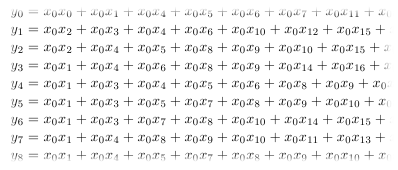## 2010-08-13

### Shmoocon 2010 Crypto Pack Solved! And Hidden Monomials!So Shmoocon 2010 challenge pack finally has a published solution! See numernia's solution here where he used MAGMA's Grobner basis facilities and jB's keygen source here where he never mentioned how the parameters of the keygen were arrived at. Of the five challenges only crypto4, the "taste of post-quantum" binary, had something new in it.

The equations you see are the most explicit way to represent MQ system (no lookup table or other type of "compression" was used as in gbe32241's work). See the HFE challenge here for a very similar setup.

I think the start of all these schemes was the MI C* scheme (see paper "Public Quadratic Polynomial-Tuples For Efficient Signature-Verification and Message-Encryption" by Matsumoto and Imai). The best explanation I've found though is probably in Koblitz's Algebraic Aspects of Cryptography.

So the main idea is that you can compute certain exponentiations in an extension field in an alternative way. First you treat the extension field elements as a vector space over the base field. This vector space has many basis to choose from, and any element can also be represented in terms of any chosen basis (the canonical one works just fine). Carefully chosen exponents of these elements resolve to linear transformations and linear expressions of the vectors. And the product of two of these special exponents resolves to a quadratic system of equation. I gave clue "Remember that an extension field is a vector space over its ground field."

The MI scheme and schemes to follow (HFE and so-on) do their best to hide this exponentiation by wrapping the expression for the exponentiation in linear transformations or adding/removing variables and equations. It's these obfuscating elements that become the private information. You didn't have to worry about any of this due to the clue: "there is no scheme in the system, no trapdoor".

So how to solve this thing? Let's treat the system like a black box. If you suspect that the black box is performing exponentiation, what's the simplest test to affirm this? Send in the identity: {1}. No matter the exponent, the identity will be returned. In the polynomial basis representation of the elements, we still don't know which direction the coefficients are listed though. No problem. With a 40-bit input, we try a 1 coefficient at either end:
```blackbox(0x8000000000); // returns 000000E0BCE7BA8A
blackbox(0x0000000001); // returns 0000000000000001```
Cool so now we know the LSB of the input is the 0'th coefficient, and it is easy to send in {1}, {x}, {x+1}, etc. But we are faced with a new problem: we don't know what reduction polynomial is used in the field computations. There is only one GF(2^40) field, but the same elements have drastically different representations depending on the reducing polynomial (math nerds talk about isomorphism here).

Can we craft some probe inputs so that the black box will yield to us its reduction polynomial? Yes!

We send element {x}. The blackbox will give us {x}^e (mod p) where e is the unknown exponent and p is the unknown reduction polynomial. We send also element {x^2} and the blackbox will return to us {x^2}^e (mod p) = {x}^(2e) (mod p).

Both of these results are already reduced (mod p). But *OUTSIDE* of the blackbox, we square the first result ourselves on the left hand side, and equate to the second result on the right hand side:
```({x}^e (mod p))^2 = {x}^(2e) (mod p)
-> p | ({x}^e (mod p))^2 - {x}^(2e)
-> p * q = ({x}^e (mod p))^2 - {x}^(2e)```
And q is just some other polynomial. So we compute the right hand side of this last equation and factor it, hoping to find some large 40-degree irreducible polynomial! Enough talk, let's do it!
```blackbox(0x0000000002 /* {x} */); // returns 0x0EFFD5DBF6 which is {x^n}
blackbox(0x0000000004 /* {x^2} */); // returns 0x0400040008 which is {x^(2n)}```
Let's flee to our CAS now, the venerable PARI/GP! We convert these results to polynomials a, b:
```a = (x^35 + x^34 + x^33 + x^31 + x^30 + x^29 + x^28 + x^27 + x^26 + x^25 + x^24 + x^23 + \
x^22 + x^20 + x^18 + x^16 + x^15 + x^14 + x^12 + x^11 + x^9  + x^8  + x^7  + x^6  + \
x^5  + x^4  + x^2  + x)*Mod(1,2)
b = (x^34 + x^18 + x^3)*Mod(1,2)```
And finally reveal our p!
```lift(factor(a*a + b))
[x 2]
[x^2 + x + 1 1]
[x^12 + x^9 + x^5 + x^4 + x^2 + x + 1 1]
[x^14 + x^13 + x^11 + x^10 + x^7 + x^6 + x^3 + x + 1 1]
[x^40 + x^38 + x^37 + x^36 + x^35 + x^33 + x^30 + x^29 + x^27 + x^26 + x^24 + x^23 + x^22 + \
x^19 + x^15 + x^14 + x^12 + x^11 + x^6 + x^5 + 1 1]```
It is the last 40-degree polynomial, converted to bit representation, it's 0x017A6DC8D861.

Finding the exponent e is simple now: you may brute outright, brute only exponents that are coprime with ord(GF*(2^40)) (else the blackbox is not a bijection), or use Coppersmith or whatever your CAS uses. It's low, just 257.

So the blackbox is doing exponentiation by 257 and field elements are represented bit-wise with the discovered polynomial. To invert, just exponentiate by d = 257^-1 (mod ord(GF*(2^40))) = 551894941568.